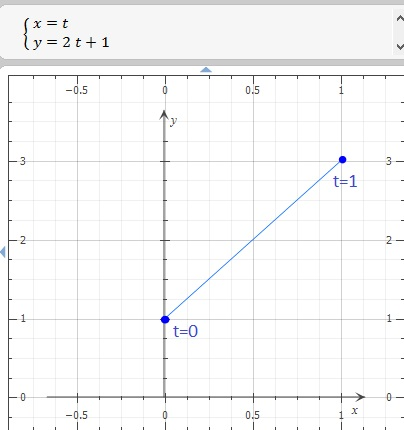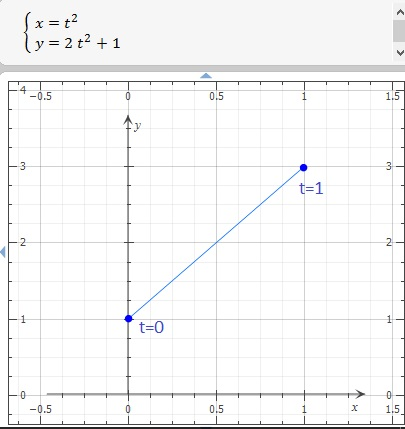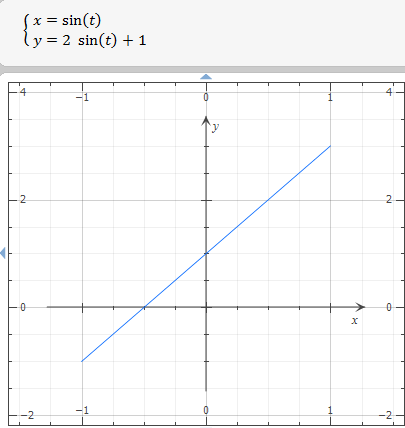# At time t minutes, robot A is at (t,2t+1) and robot B is at t^2,2t^2+1) a. Where is each robot...

## Question:

At time {eq}t {/eq} minutes, robot {eq}A {/eq} is at {eq}(t,2t+1) {/eq} and robot {eq}B {/eq} is at {eq}(t^2,2t^2+1) {/eq}

a. Where is each robot when {eq}t=0 {/eq} and {eq}t=1 {/eq}?

b. Sketch the path each robot follows during the first minute.

c. Find the slope of the tangent line {eq}\frac {dy}{dx} {/eq}, to the path of each robot at {eq}t=1 {/eq} minute.

d. Find the speed of each robot at {eq}t=1 {/eq} minute

e. Discuss the motion of a robot which follows the path {eq}(\sin(t), 2 \sin(t)+1) {/eq} for {eq}20 {/eq} minutes.

## Parametric Functions

If we have an x-axis position versus time and a y-axis position versus time function, we have a parametric function, where the parameter is the time. Also, we can find their speed function with differentiation.

a Where is each robot when {eq}t=0 \; \text{ & } \; t=1 {/eq}

Robot A:

Position vs time functions are: {eq}\displaystyle x(t)=t \; \text{ & } \;y(t)=2t+1 \\ {/eq}

So, x-axis position when {eq}t=0 {/eq} is:

{eq}\displaystyle x(0)=0 {/eq}

And, y-axis position when {eq}t=0 {/eq} is:

{eq}\displaystyle y(0)=2(0)+1 \\ \displaystyle y(0)=1 \\ {/eq}

The robot's position at {eq}t=0 {/eq} is {eq}\displaystyle (0,1) {/eq}

And, for {eq}t=1 \\ \displaystyle x(1)=1 \; \text{ & } \; y(1)=2(1)+1 \\ \displaystyle (1,3) \\ {/eq}

Robot B:

{eq}\displaystyle x(t)=t^2 \; \text{ & } \; y(t)=2t^2+1 \\ \; \text{ for } \; t=0 \\ \displaystyle x(0)= (0)^2 \; \text{ & } \; y(0)= 2(0)^2+1 \\ \displaystyle (0,1) \\ \; \text{ & } \; \\ \; \text{ for } \; t=1 \\ \displaystyle x(1)= (1)^2 \; \text{ & } \; y(1)= 2(1)^2+1 \\ \displaystyle (1,3) \\ {/eq}

b. Sketch the path each robot follows during the first minute.

Robot A:Robot Bc. Find the slope of the tangent line {eq}\frac {dy}{dx} {/eq}, to the path of each robot at {eq}t=1 {/eq} minute.

Robot A

{eq}\displaystyle \frac{ dy }{ dx } \; = \; \frac{ dy/dt }{ dx/dt } \\ {/eq}

So,

{eq}\displaystyle \frac{dy}{dt} \left[ 2t+1 \right] = 2 \\ \; \text{ & } \; \\ \displaystyle \frac{dx}{dt} \left[ t \right] = 1 \\ {/eq}

Therefore, {eq}\displaystyle \frac{ dy/dt }{ dx/dt } \; = \; \frac{ 2 }{ 1 } \\ \displaystyle \frac{ dy }{ dx } \; = \; 2 \\ {/eq}

Therefore, the slope of the tangent line at {eq}t=1 {/eq} is:

{eq}\displaystyle 2 {/eq}

Robot B

{eq}\displaystyle \frac{dy}{dt} \left[ t^2 \right] = 2t \\ \; \text{ & } \; \\ \displaystyle \frac{dx}{dt} \left[ 2t^2+1 \right] = 4t \\ {/eq}

Therefore, {eq}\displaystyle \frac{ dy/dt }{ dx/dt } \; = \; \frac{ 2t }{ 4t } \\ \displaystyle \frac{ dy }{ dx } \; = \; 1/2 \\ {/eq}

Therefore, the slope of the tangent line at {eq}t=1 {/eq} is:

{eq}\displaystyle 1/2 {/eq}

d. Find the speed of each robot at {eq}t=1 {/eq} minute

Speed vs time is given by {eq}\displaystyle \frac{d}{dt} \left[ x(t) \right]= v_x(t) \; \text{ & } \; \frac{d}{dt} \left[ y(t) \right]= v_y(t) \\ {/eq}

Robot A:

{eq}\displaystyle v_x(t)=1 \; \text{ & } \; v_y(t)=2 \\ {/eq}

Then for {eq}t=1 \\ \displaystyle v_x(1)=1 \; \text{ & } \; v_y(1)=2 \\ {/eq}

Robot B:

{eq}\displaystyle v_x(t)=2t \; \text{ & } \; v_y(t)=4t \\ {/eq}

Then for {eq}t=1 \\ \displaystyle v_x(1)=2(1) \; \text{ & } \; v_y(1)=4(1) \\ \displaystyle v_x(1)=2 \; \text{ & } \; v_y(1)=4 \\ {/eq}

e. Discuss the motion of a robot which follows the path {eq}(\sin(t), 2 \sin(t)+1) {/eq} for {eq}20 {/eq} minutes.

Trigonometric functions have repetitive behavior, for example, the function {eq}\displaystyle x(t)= \sin(t) {/eq} its range is {eq}x \; \in \; (-1,1) \; \text{ or } \; -1 \; \leq \; x \; \leq \; 1 \\ {/eq}

Therefore, the Robot has a repetivite path, please see the graph: